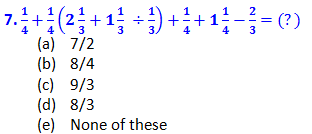# Quantitative Aptitude Practice Questions for IBPS Clerk – Set 1

Quantitative Aptitude Practice Questions for IBPS Clerk – Set 1
Directions (1 – 5): What will come in place of the question mark (?) in the following number series?
1. 30,   18,   10,   7.5,   ?,   8.25
(a)  9
(b)  7.4
(c)  18
(d)  8.25
(e)  7.5
2. 11,  21,  48,  97,  178,  ?
(a)  369
(b)  314
(c)  307
(d)  316
(e)  306
3. 0.5, 1.5, 9, 81, ?
(a)  919
(b)  974
(c)  960
(d)  916
(e)  972
4. 5   12   39   160   805   (?)
(a)  4898
(b)  4890
(c)  5276
(d)  4836
(e)  None of these
5. 3    12   (?)   72   135   228   357
(a)  33
(b)  24
(c)  36
(d)  28
(e)  None of these
Directions (6 – 15): What should come in place of question mark in the following question?
6.  (15967 – 12437 – 1910) ÷ ? = 27
(a)  60
(b)  50
(c)  65
(d)  45
(e)  None of these8. 28 × 104 ÷ (28 – 21 + 6) + 3 = ?
(a)  227
(b)  204
(c)  224
(d)  228
(e)  23410.  (43% of 27500) – (38% of 29900) = ?
(a)  493
(b)  447
(c)  436
(d)  463
(e)  None of these
11. [(130)÷ 25 × 15] ÷ 30 = ?
(a)  352
(b)  314
(c)  326
(d)  338
(e)  None of these
12. (1/9)0 + (9)0 + (343)-1/3 + (36)3/2
(a)  323/8
(b)  1829/7
(c)  1527/7
(d)  7/1829
(e)  7/1527
13. 75% of 8523 + 20% of 6245 = ?
(a)  8042.21
(b)  8136.28
(c)  8625.35
(d)  8220.19
(e)  None of theseSolutions:
1. E) The pattern of given series is:
→ 30 ÷ 2 + 3 = 15 + 3 = 18,
→ 18 ÷ 3 + 4 = 6 + 4 = 10,
→ 10 ÷ 4 + 5 = 2.5 + 5 = 7.5,
→ 7.5 ÷ 5 + 6 = 1.5 + 6 = 7.5,
→ 7.5 ÷ 6 + 7 = 1.25 + 7 = 8.25,
? = 7.5
2. E) The pattern of given series is:
→  11          21           48              97             178         ?
+10        +27          +49            +81
+17           +22           +32
+5          +10              ?
? = 15 + 32 + 81 + 178
=  306
3. E) The pattern of given series is:
→ 0.5 × 3 = 1.5,
→ 1.5 × 6 = 9,
→ 9 × 9 = 81,
​81 × 12 = 972
? = 972
4. D) The pattern of given series is:
→ 5,
→ 12 = 5 × 2 + 2,
→ 39 = 12 × 3 + 3,
→ 160 = 39 × 4 + 4,
→ 805 = 160 × 5 + 5,
→ ? = 805 × 6 + 6,
5. A) The pattern of given series is:
→ 3 = 13 + 2
→ 12 = 23 + 4,
→ ? = 33 + 6 = 27 + 6,
→ ? = 33
→ 72 = 43 + 8,
→ 135 = 53 + 10,
(6 – 10): Common Explanation:
Follow BODMAS rule to solve this question, as per the order given below,
Step-1-Parts of an equation enclosed in 'Brackets' must be solved first,
Step-2-Any mathematical 'Of' or 'Exponent' must be solved next,
Step-3-Next, the parts of the equation that contain 'Division' and 'Multiplication' are calculated,
Step-4-Last but not least, the parts of the equation that contain 'Addition' and 'Subtraction' should be calculated.
6. A)   7. D)   8. A)   9. A)   10. D)   11. D)   12. C)   13. E)   14. B)   15. C)